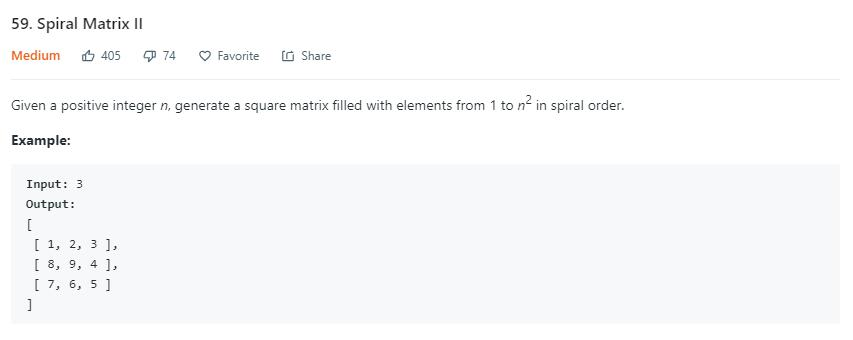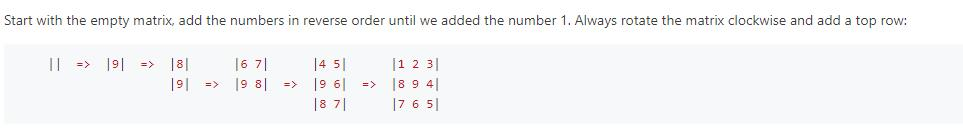# 题目描述（中等难度）54题 差不多，54 题按照螺旋状遍历，这个是按照螺旋状生成二维数组。

# 解法一

/*
* direction 0 代表向右, 1 代表向下, 2 代表向左, 3 代表向上
*/
public int[][] generateMatrix(int n) {
int[][] ans = new int[n][n];
int start_x = 0, start_y = 0, direction = 0, top_border = -1, // 上边界
right_border = n, // 右边界
bottom_border = n, // 下边界
left_border = -1; // 左边界
int count = 1;
while (true) {
// 全部遍历完结束
if (count == n * n + 1) {
return ans;
}
// 注意 y 方向写在前边，x 方向写在后边
ans[start_y][start_x] = count;
count++;
switch (direction) {
// 当前向右
case 0:
// 继续向右是否到达边界
// 到达边界就改变方向，并且更新上边界
if (start_x + 1 == right_border) {
direction = 1;
start_y += 1;
top_border += 1;
} else {
start_x += 1;
}
break;
// 当前向下
case 1:
// 继续向下是否到达边界
// 到达边界就改变方向，并且更新右边界
if (start_y + 1 == bottom_border) {
direction = 2;
start_x -= 1;
right_border -= 1;
} else {
start_y += 1;
}
break;
case 2:
if (start_x - 1 == left_border) {
direction = 3;
start_y -= 1;
bottom_border -= 1;
} else {
start_x -= 1;
}
break;
case 3:
if (start_y - 1 == top_border) {
direction = 0;
start_x += 1;
left_border += 1;
} else {
start_y -= 1;
}
break;
}
}

}


# 解法二